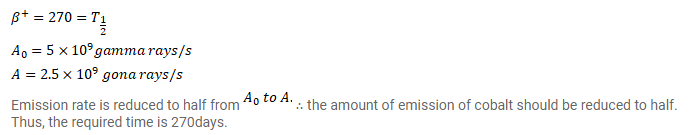# 57Co decays to 57Fe by ????+-emission. The resulting 57Fe is in its excited

Question:

${ }^{57}$ Co decays to ${ }^{57} \mathrm{Fe}$ by $\beta^{+}$-emission. The resulting ${ }^{57} \mathrm{Fe}$ is in its excited state and comes to the ground state by emitting $\gamma$ rays. The half-life of $\beta^{+}$-decays is 270 days and that of the $\gamma$ emission is $10^{-8} \mathrm{~s}$. A sample of ${ }^{57}$ Co gives $5.0 \times 10^{9} \mathrm{gamma}$ rays per second. How much time will elapse before the emission rate of gamma rays drops to $2.5 \times 10^{9}$ per second?

Solution: